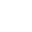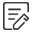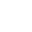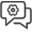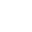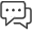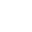Date:2022-06-28 14:48:06

# What is DB to DBI Calculator

DBi and dBd are units of power gain, both are relative values, but the reference is not the same. The reference reference for dBi is an omnidirectional antenna; the reference reference for dBd is a dipole.

It is generally believed that dBi and dBd represent the same gain, and the value expressed in dBi is 2.15 larger than the value expressed in dBd. (ie dBi=dBd+2.15). G(dBi)=10lgGi G(dBd)=10lgGd

DBm is a value that evaluates the absolute value of power, and the calculation formula is: 10lgP (power value/1mw).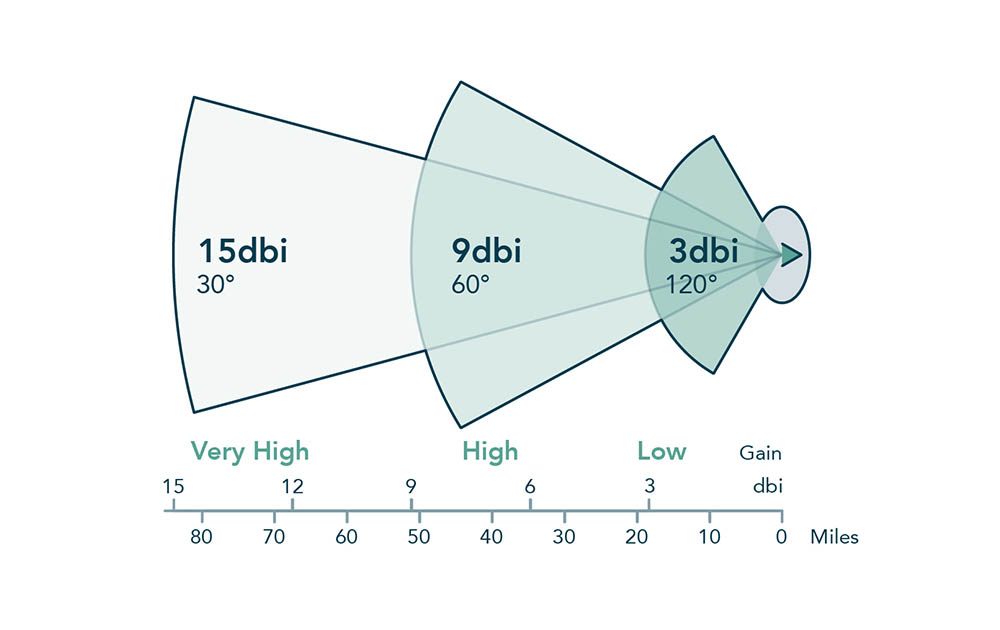1. Db to dbi calculator

1.1 If the transmit power P is 1mw, it is 0dBm after converting to dBm.

1.2 For 40W power, the converted value in dBm should be:

10lg(40W/1mw)=10lg(40000)=10lg4+10lg10+10lg1000=46dBm.

1.3 For an antenna with a gain of 16dBd, when its gain is converted into dBi, it is 18.15dBi (generally ignoring the decimal place, it is 18dBi).

1.4 0dBd=2.15dBi.

1.5 GSM900 antenna gain can be 13dBd (15dBi), GSM1800 antenna gain can be 15dBd (17dBi).

2. Db to dbi calculator extended information:

2.1 Take power as an example:

Signal power is X = 100000W = 10^5

The reference power is Y=1W

The value of dB: Lx(dB) = 10*lg(10^5W/1W) dB= 10*lg(10^5) dB= 50 dB

2.2 Similarly:

X = 10^-15

Lx(dB) = 10*lg(X) dB= 10*lg(10^-15) dB= -150 dB

Generally speaking, in engineering, there is only addition and subtraction between dB and dB, no multiplication and division. The most commonly used is subtraction.

DBm minus dBm is actually the division of two powers, and the division of signal power and noise power is the signal-to-noise ratio (SNR). For example: 30dBm - 0dBm = 1000mW/1mW = 1000 = 30dBm.

DBm plus dBm is actually the multiplication of two powers and has no actual physical meaning.

Like all "units" that take logarithms, decibels have: the smaller the value, the easier it is to read and write; the operation is convenient; it conforms to the sense of hearing and is easy to estimate.

-3dB is also called the half power point or cutoff frequency point. At this time, the power is half of the normal time, and the voltage or current is 1/√2 of the normal time. 【∵P=I^2*R or P=U^2/R and 10^0.1505=√2】

0dB means that the output is as large as the input or both comparison signals.

Simply put, dB is a ratio. For example, in the audio industry, doubling the power is 3dB.

The meaning and relationship between dBm, dBw, dBu, dBc

DBm is a value that examines the absolute value of power. The calculation formula is: 10lgP (power value/1mw), which is an absolute value, and 0dBm is the energy converted by 1 milliwatt.

Like dBm, dBw is a unit that represents the absolute value of power (it can also be considered as a ratio based on 1W power), and the calculation formula is: 10lg (power value/1w).

The conversion relationship between dBw and dBm is: 0 dBw = 10lg1 W = 10lg1000 mw = 30 dBm, it can be seen that 0dBw is a much larger unit than 0dBm, and the power difference is 1000 times, so professional audio equipment , the most typical example is the power amplifier, the 0dB scale is the maximum value, and the knob of the power amplifier is actually an attenuator;

DBu is a unit parameter with a voltage of .775v as a reference value, and dBv is a reference value of 1V. Therefore, 0dBv is approximately equal to 2.2dBu; their conversion formula is: x dBv = (x + 2.2)dBu

DBc is more common in digital audio systems, and it is also a value that considers relative power. For example, 0dBc set inside a processor is actually equivalent to -24dBm.